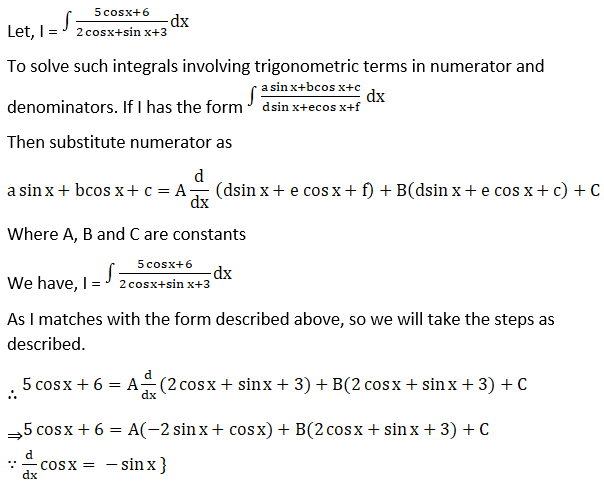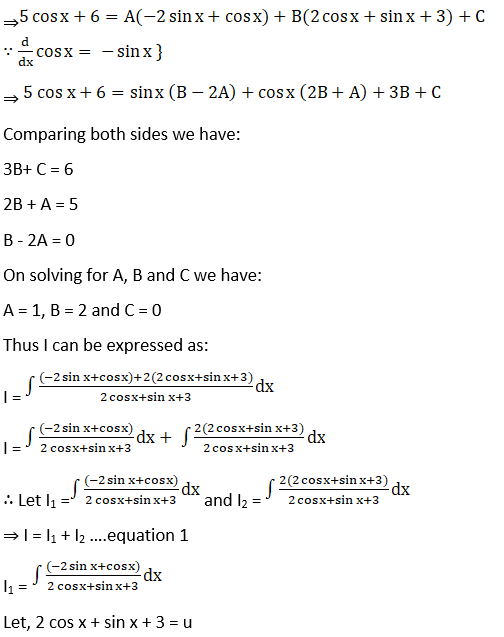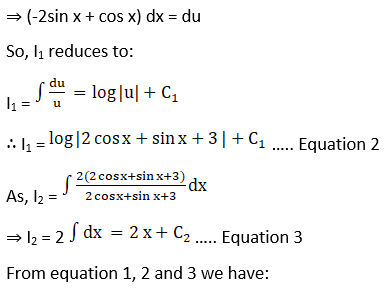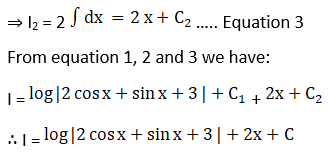# RD Sharma Solutions For Class 12 Maths Exercise 19.24 Chapter 19 Indefinite Integrals

RD Sharma Solutions for Class 12 Maths Exercise 19.24 Chapter 19 Indefinite Integrals is provided here. The subject experts at BYJU’S outline the concepts in a clear and precise manner, based on the IQ level of students. Our solution module utilizes numerous shortcut tips and practical examples to explain all the exercise questions in a simple and easily understandable language. If you wish to obtain an excellent score, solving RD Sharma Class 12 Solutions is a must.

Here, students will learn alternative technique to determine integral of the form

$$\begin{array}{l}\int \frac{1}{\sqrt{a \sin x+b \cos x}}\ dx, \int \frac{1}{a+b \sin x}\ dx\end{array}$$
. The solutions to all questions in RD Sharma books are given here in a detailed and step by step way to help students understand more effectively. The PDF of RD Sharma Solutions for Class 12 Maths Chapter 19 Exercise 19.24 are provided here.

## Download the PDF of RD Sharma Solutions For Class 12 Chapter 19 – Indefinite Integrals Exercise 19.24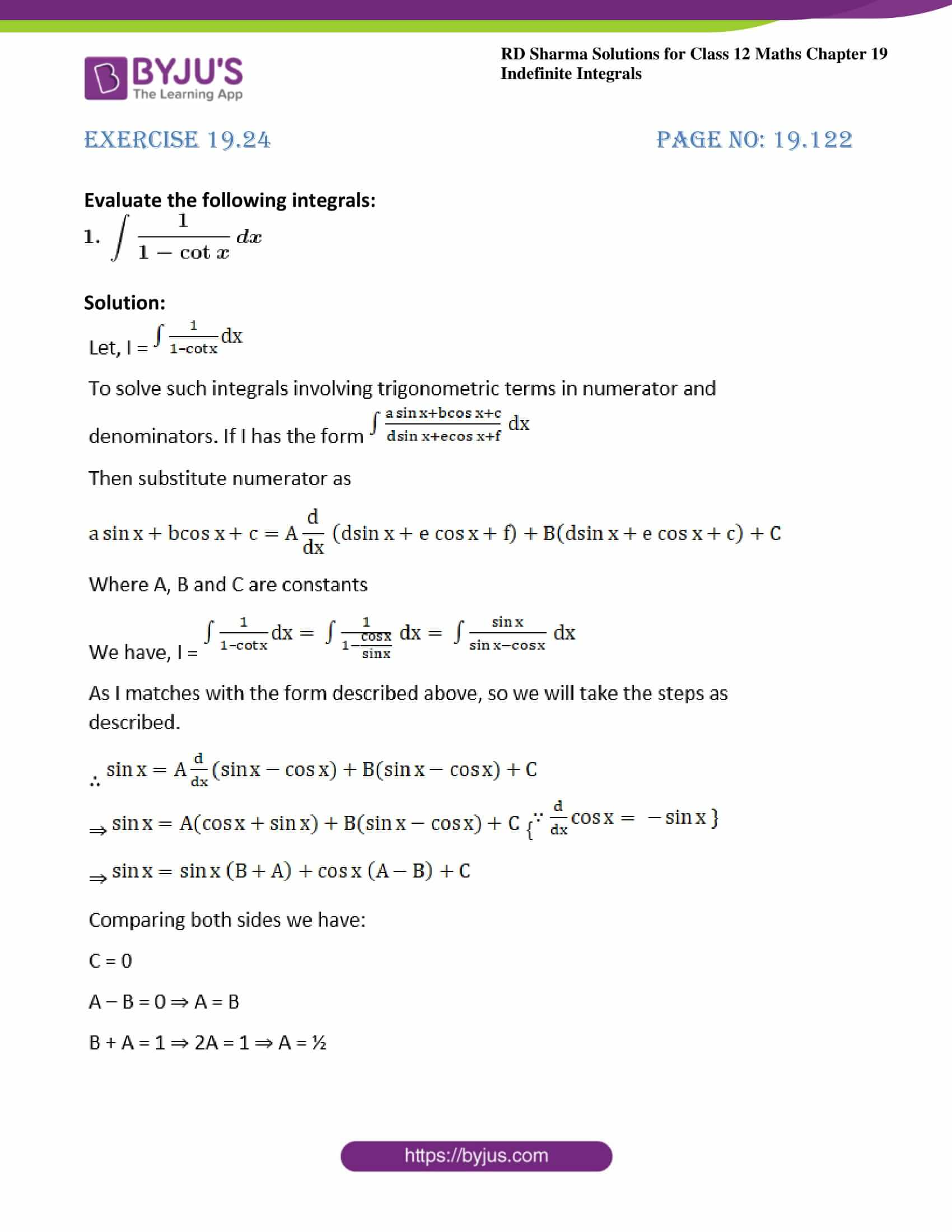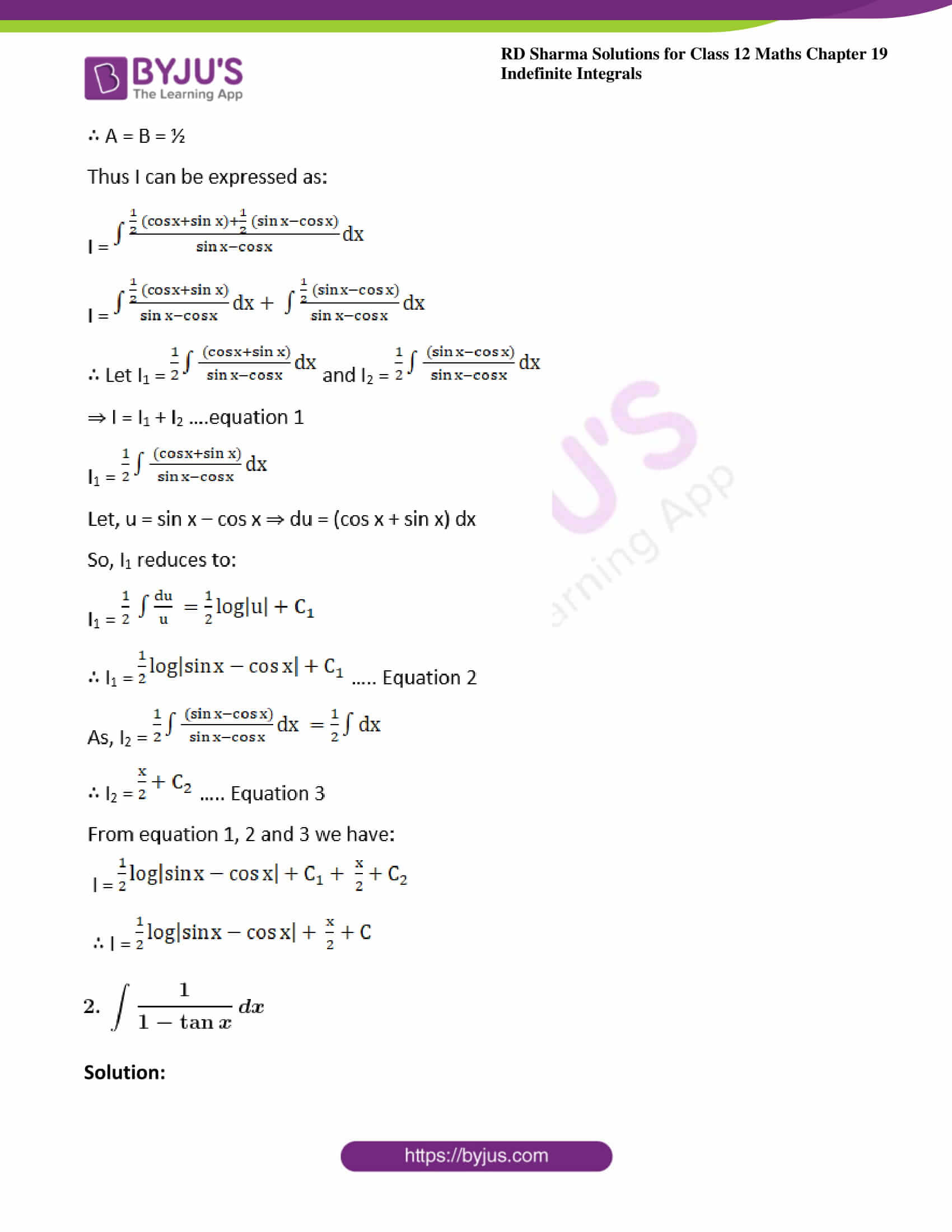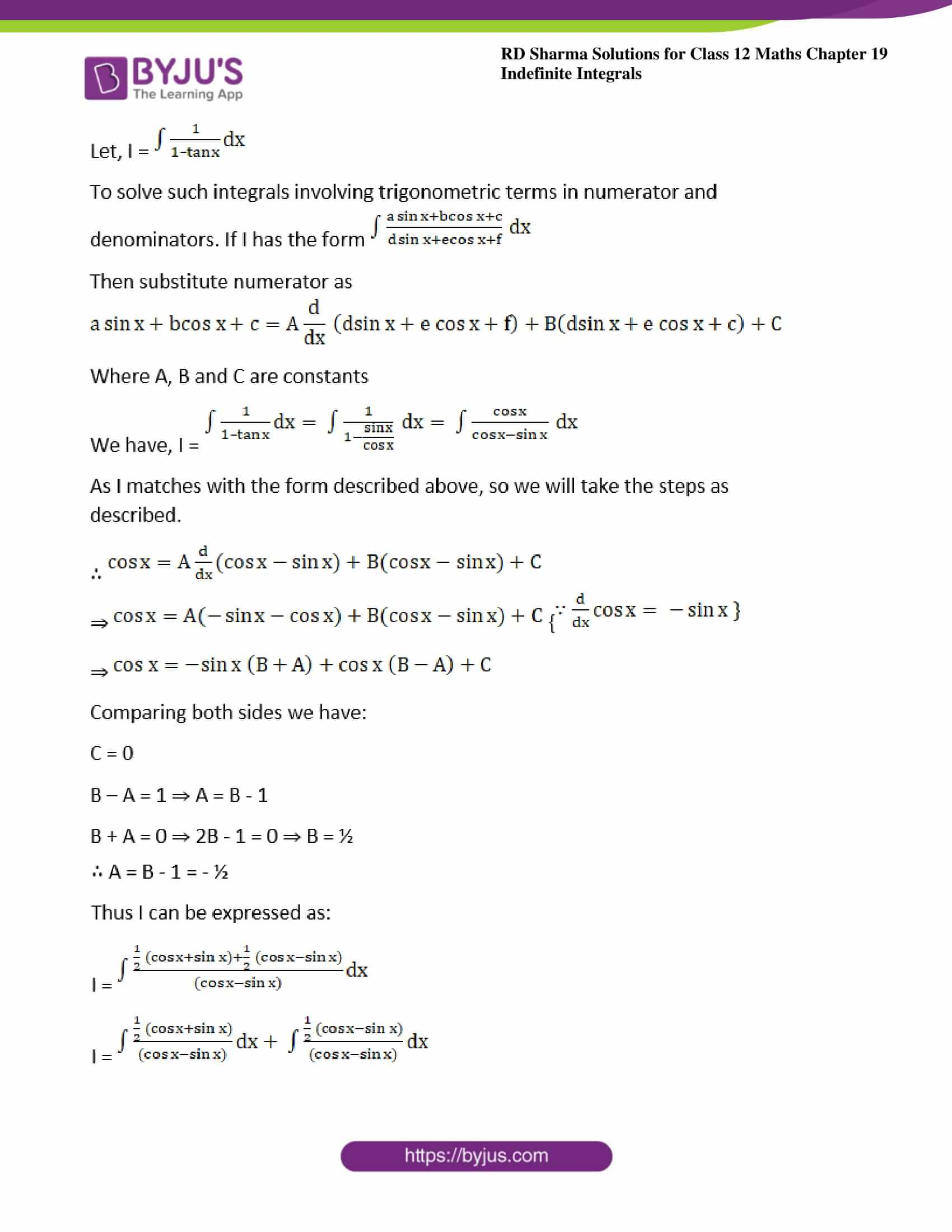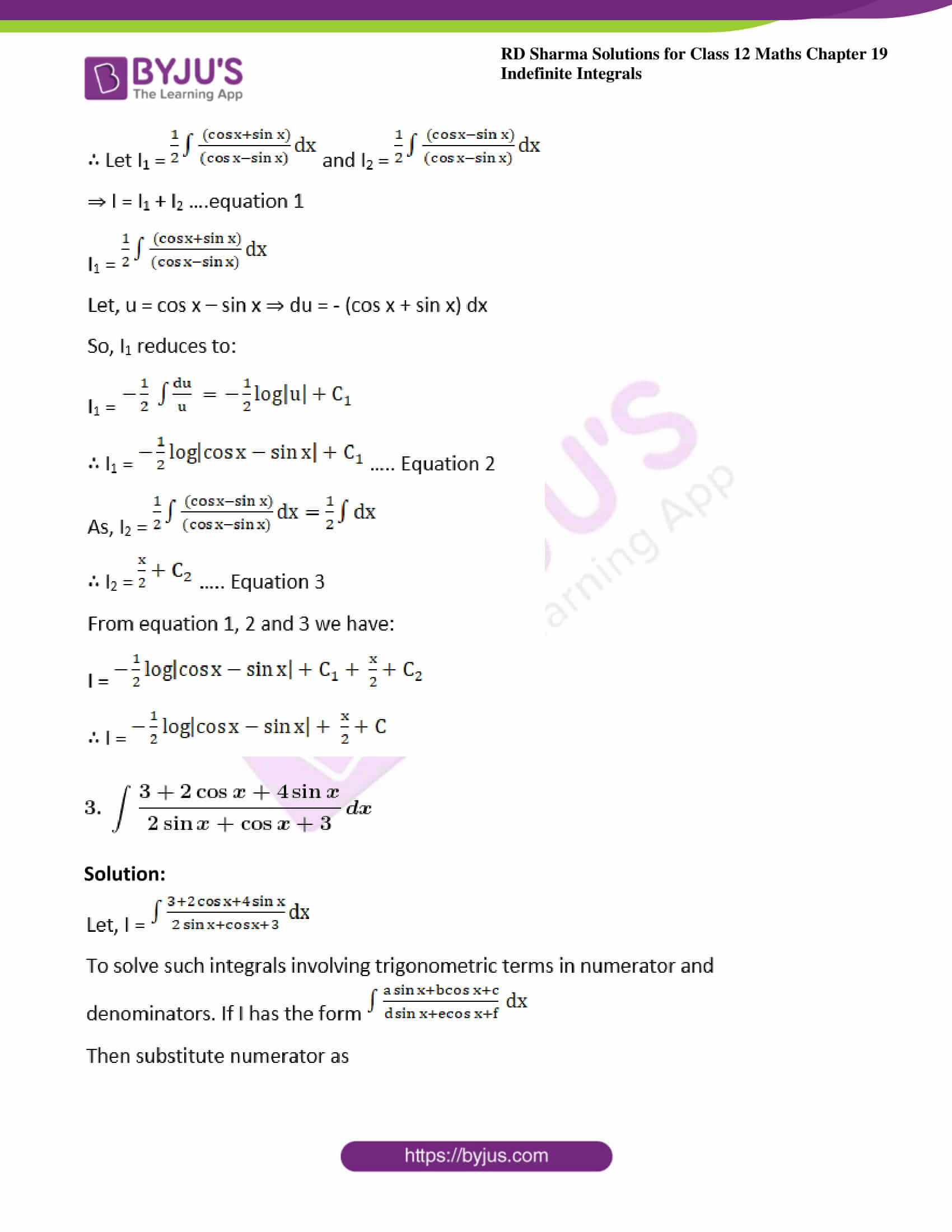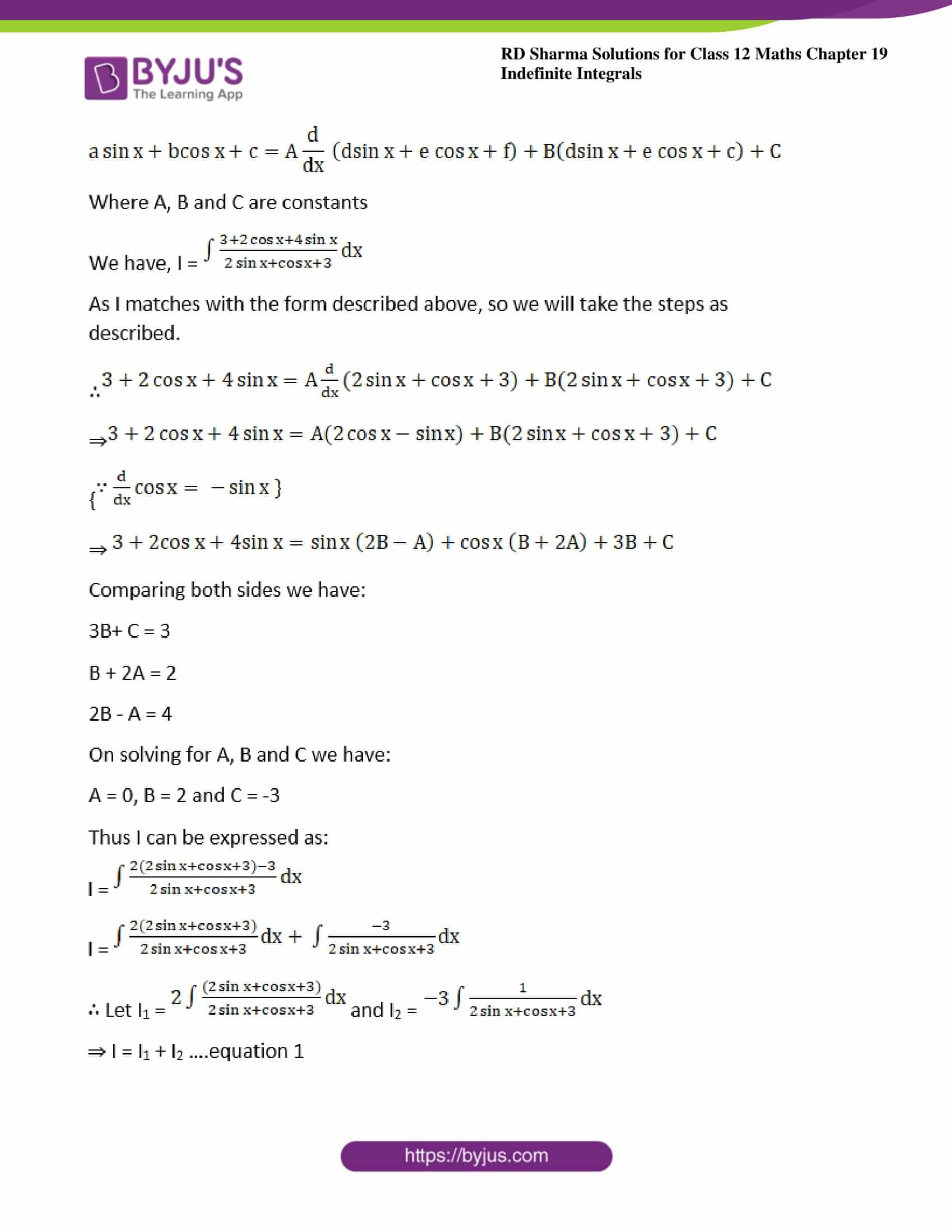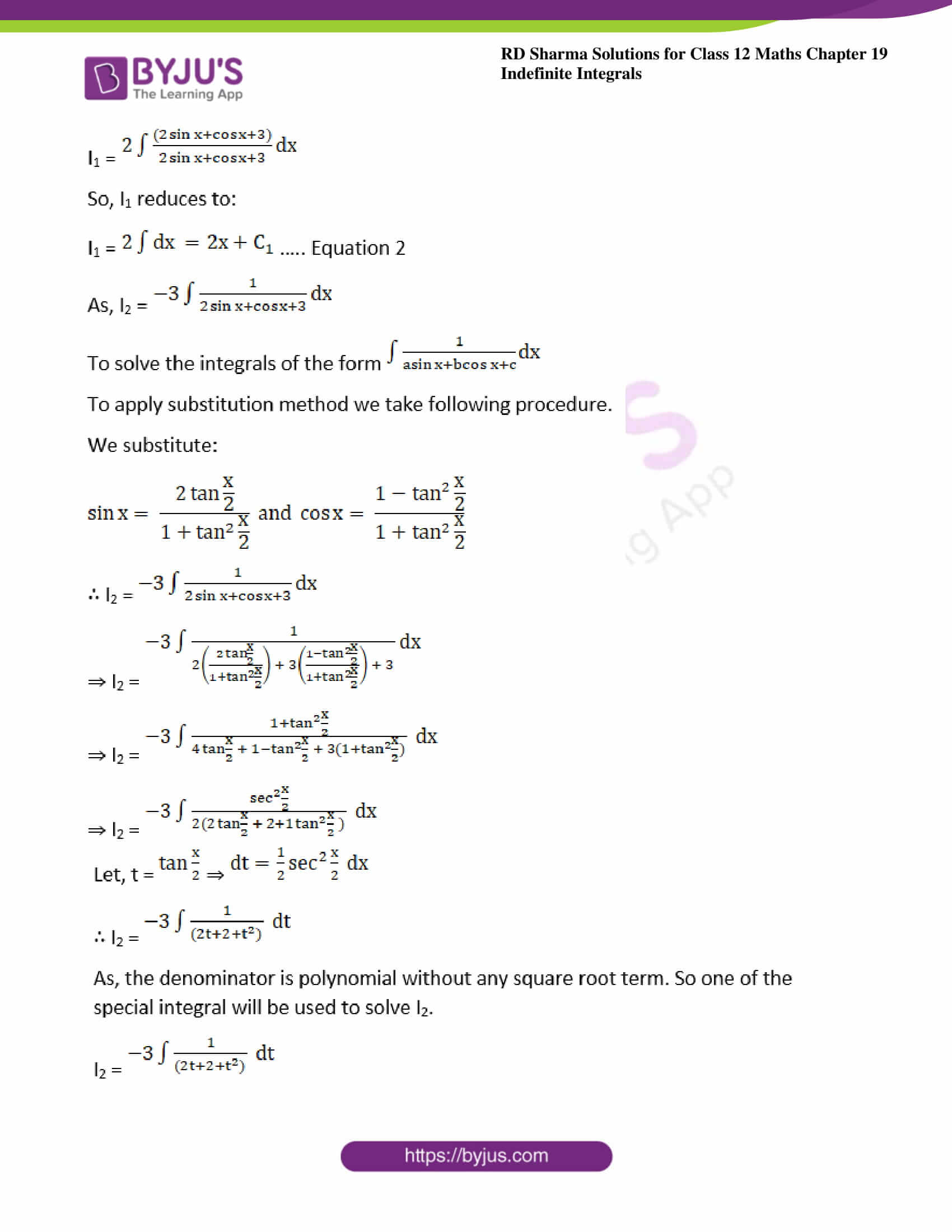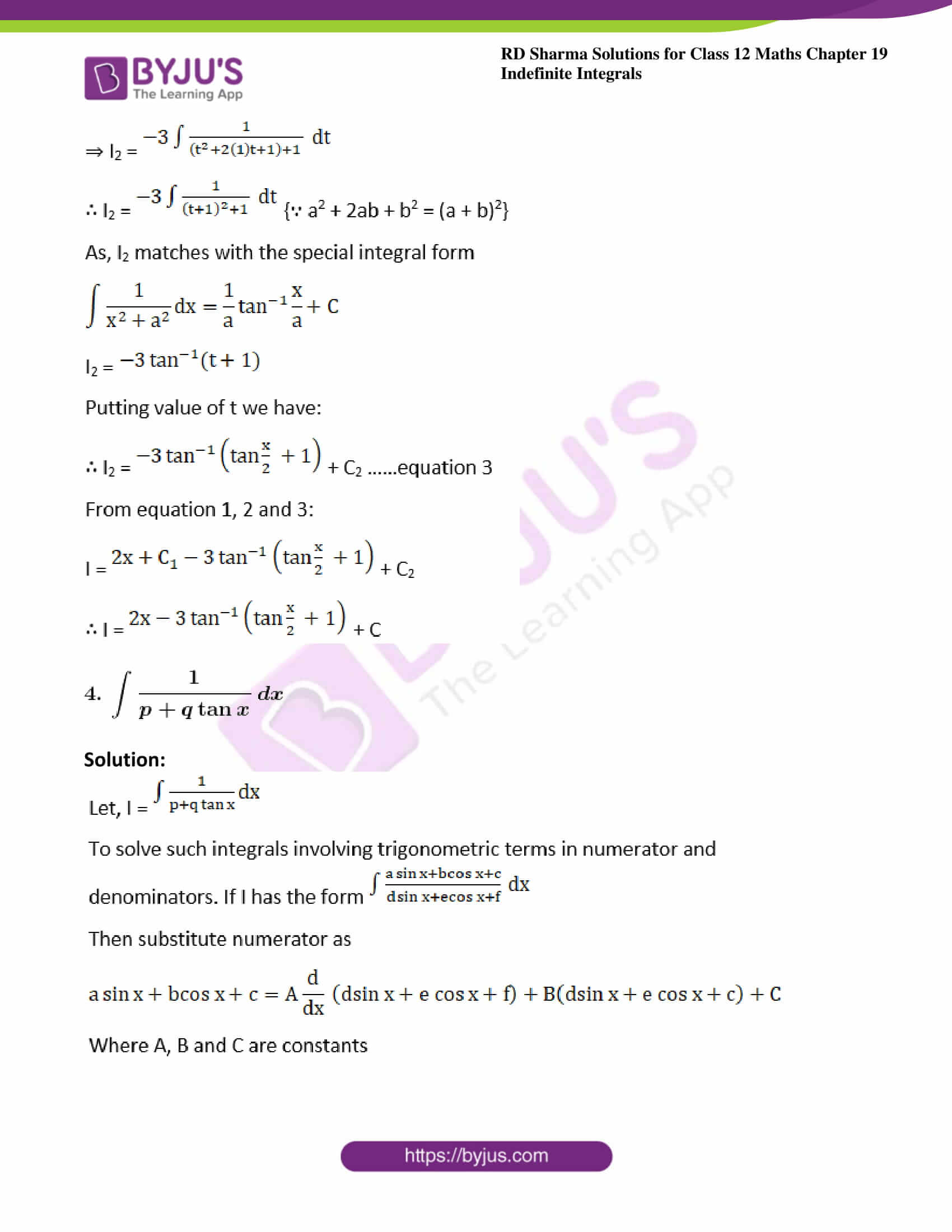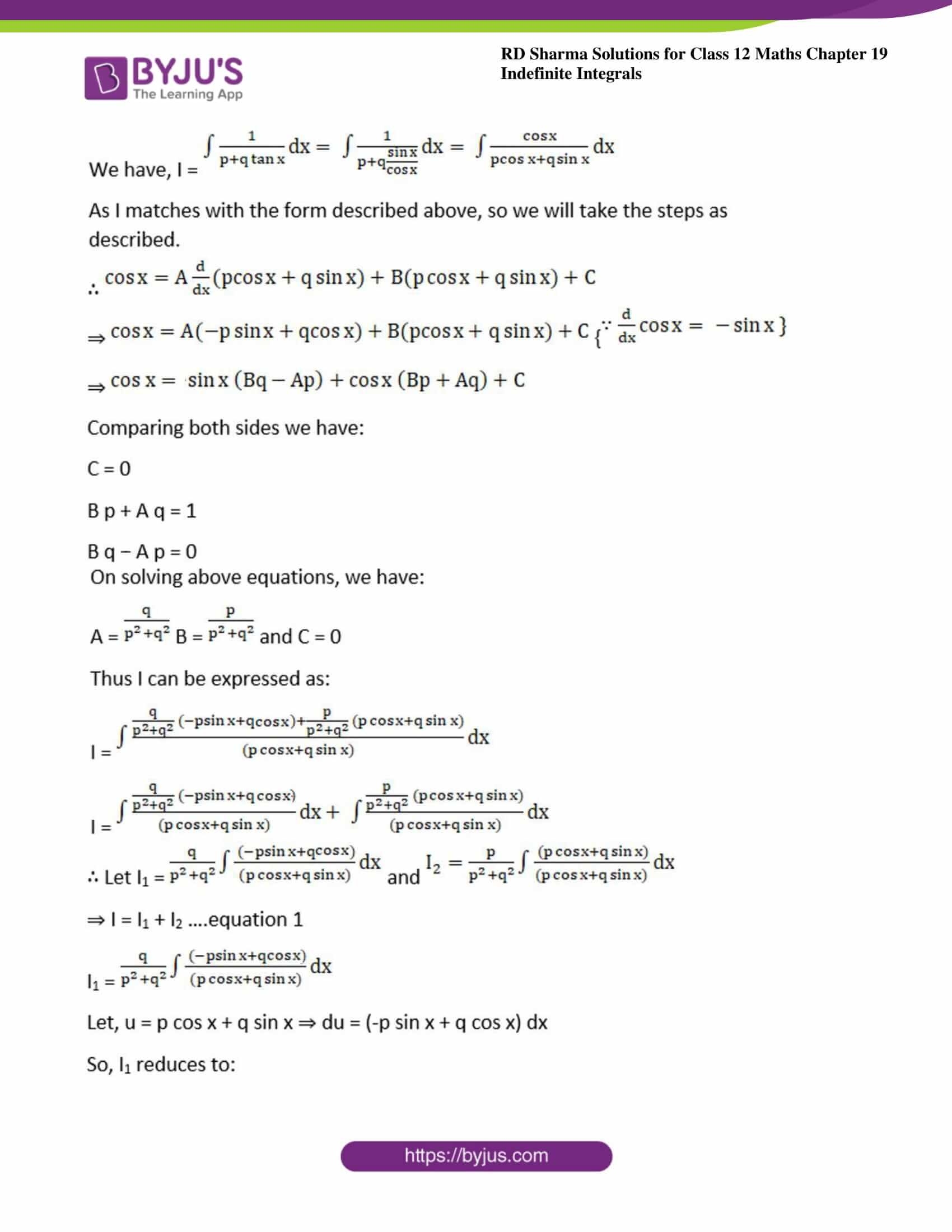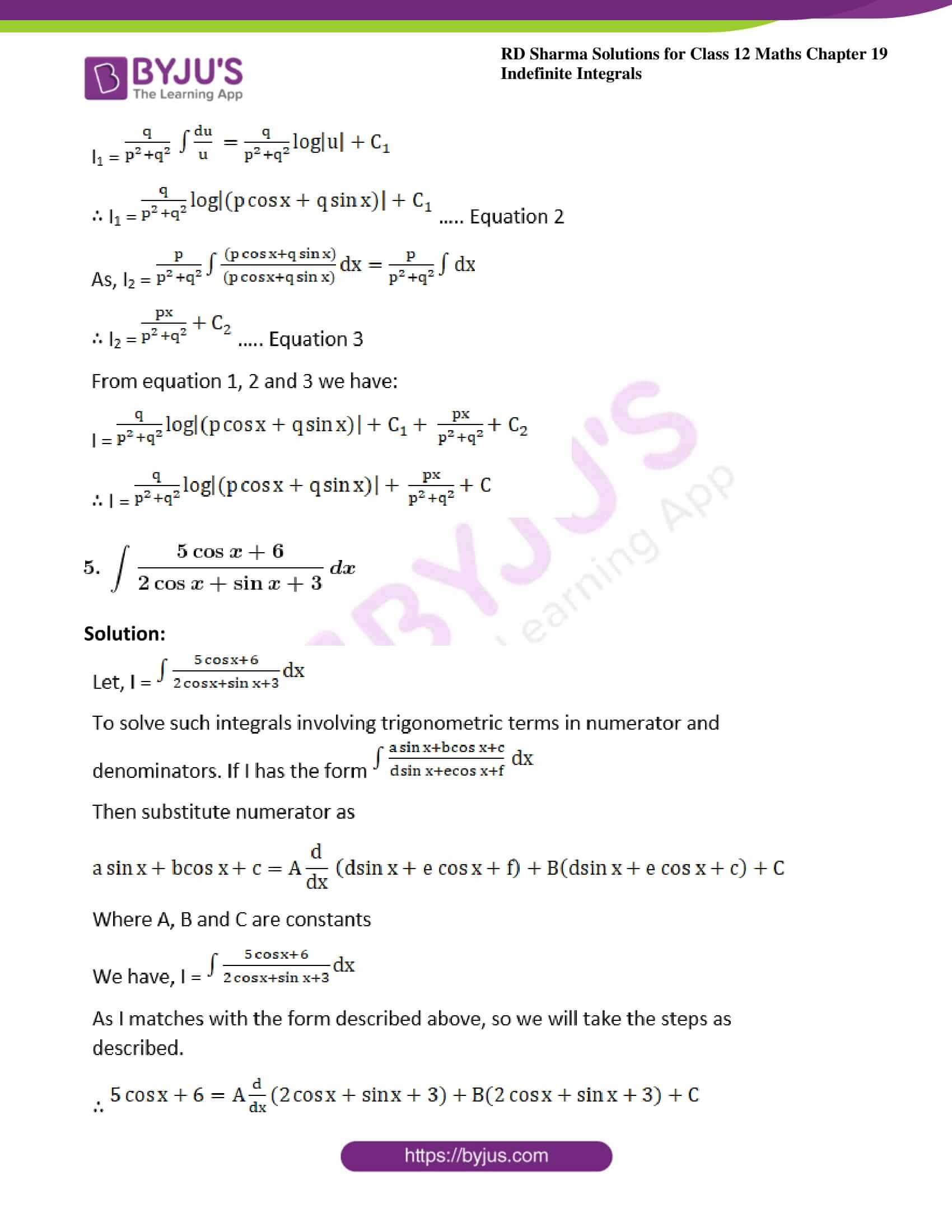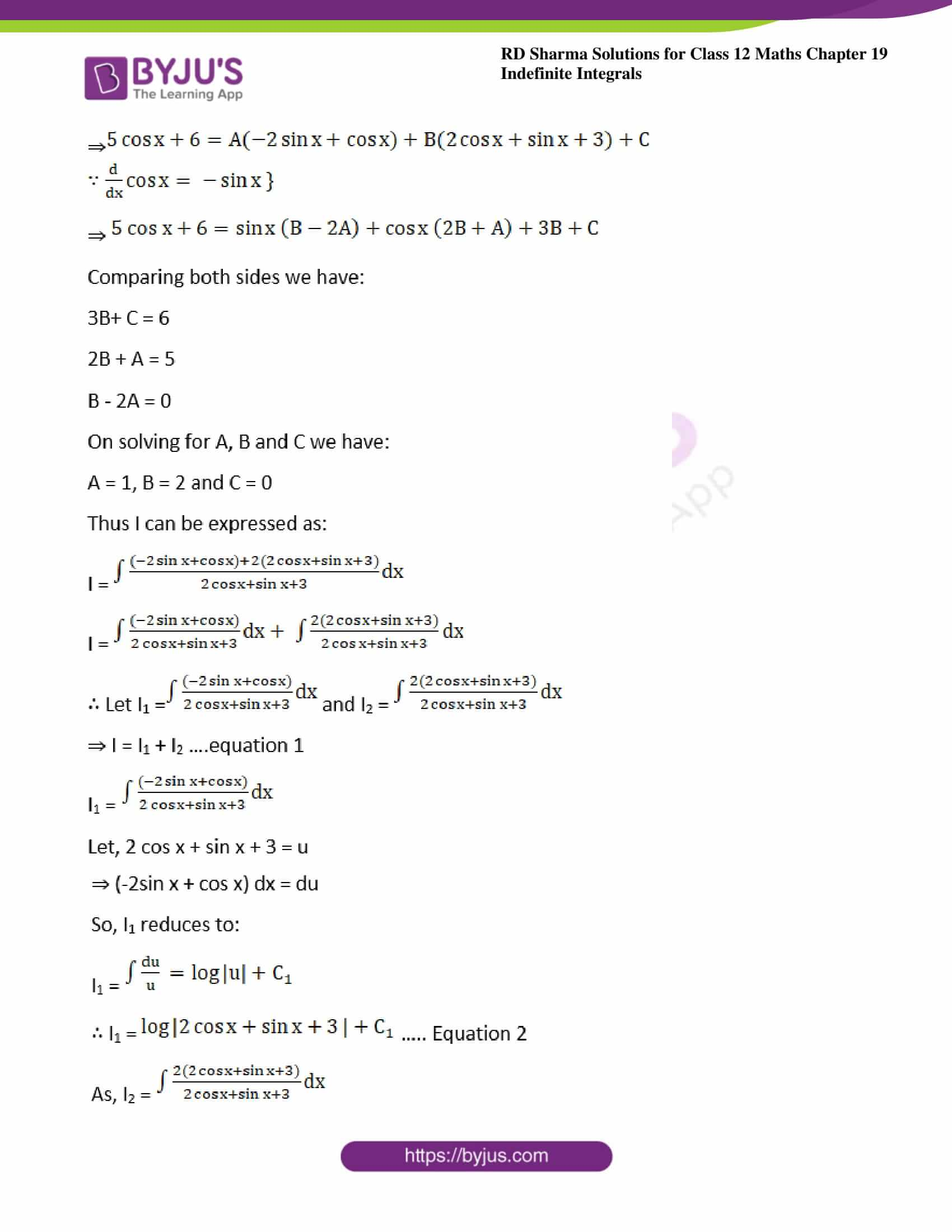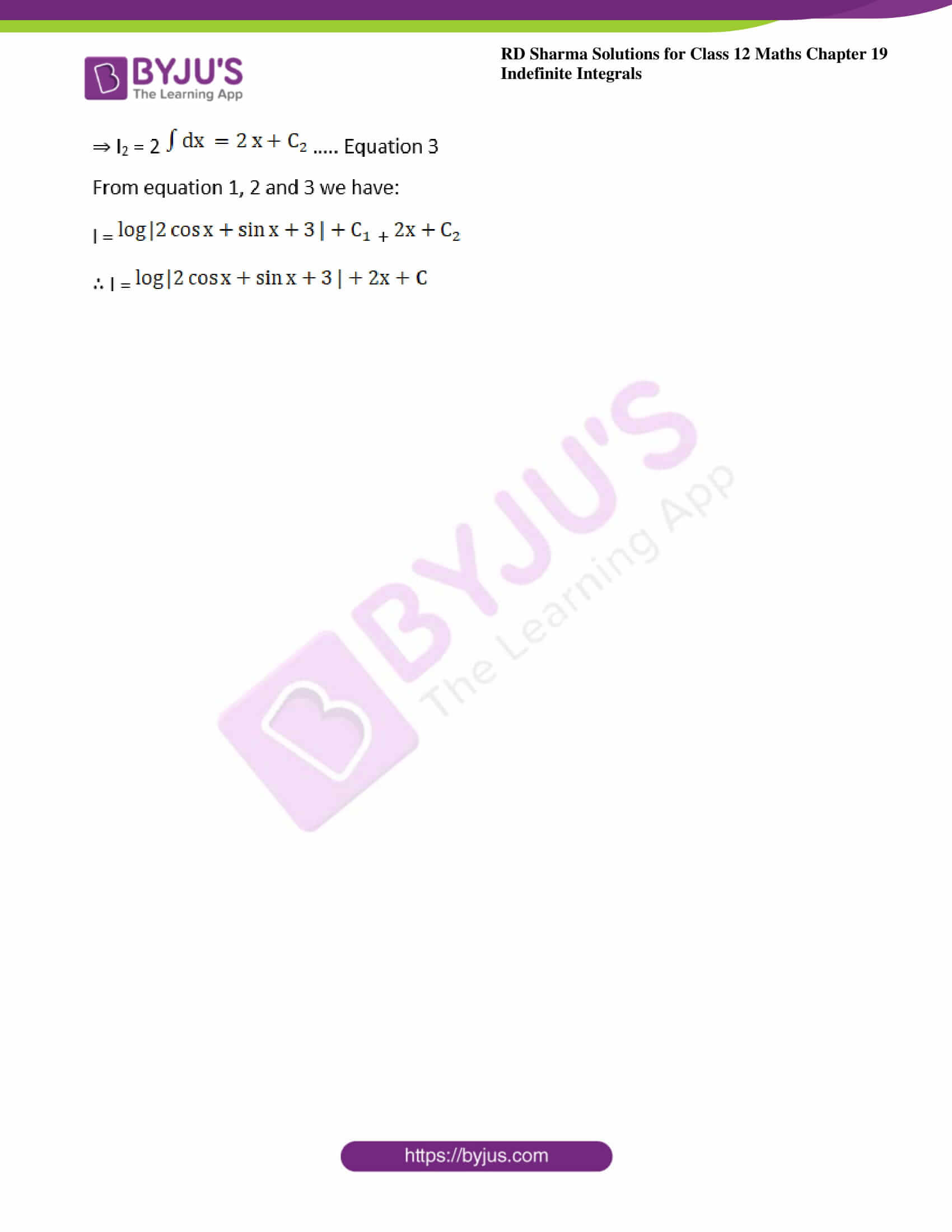### Access other exercises of RD Sharma Solutions For Class 12 Chapter 19 – Indefinite Integrals

Exercise 19.1 Solutions

Exercise 19.2 Solutions

Exercise 19.3 Solutions

Exercise 19.4 Solutions

Exercise 19.5 Solutions

Exercise 19.6 Solutions

Exercise 19.7 Solutions

Exercise 19.8 Solutions

Exercise 19.9 Solutions

Exercise 19.10 Solutions

Exercise 19.11 Solutions

Exercise 19.12 Solutions

Exercise 19.13 Solutions

Exercise 19.14 Solutions

Exercise 19.15 Solutions

Exercise 19.16 Solutions

Exercise 19.17 Solutions

Exercise 19.18 Solutions

Exercise 19.19 Solutions

Exercise 19.20 Solutions

Exercise 19.21 Solutions

Exercise 19.22 Solutions

Exercise 19.23 Solutions

Exercise 19.25 Solutions

Exercise 19.26 Solutions

Exercise 19.27 Solutions

Exercise 19.28 Solutions

Exercise 19.29 Solutions

Exercise 19.30 Solutions

Exercise 19.31 Solutions

Exercise 19.32 Solutions

### Exercise 19.24 Page No: 19.122

Evaluate the following integrals: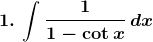Solution: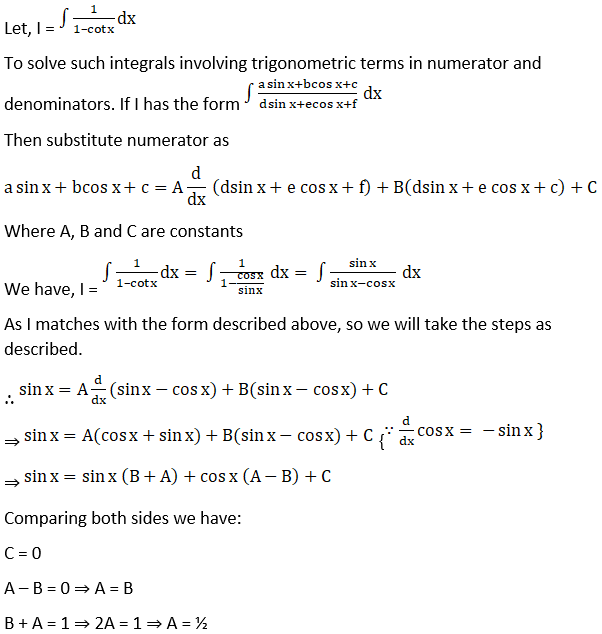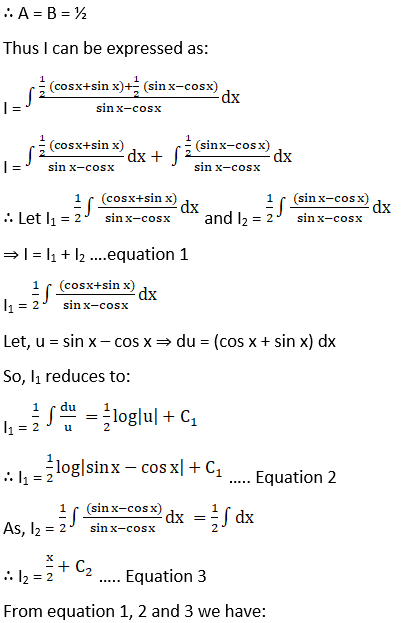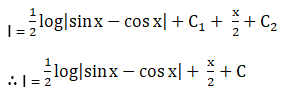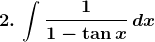Solution: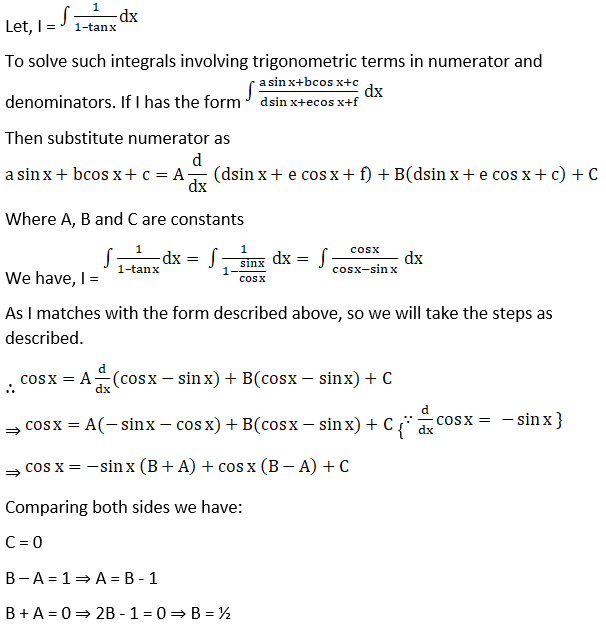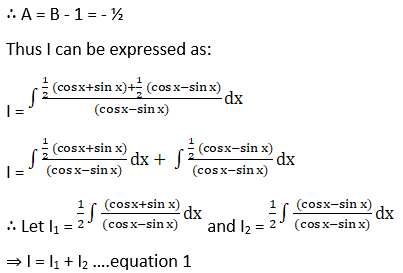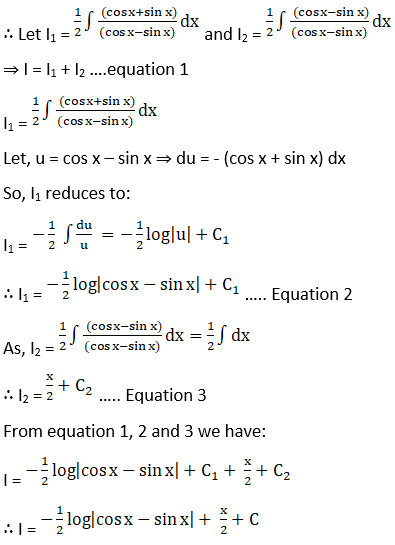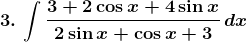Solution: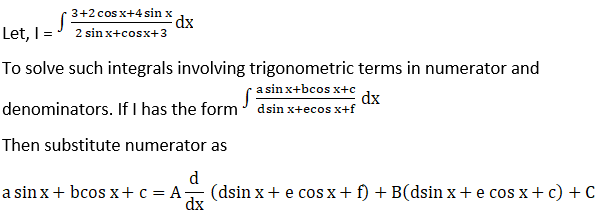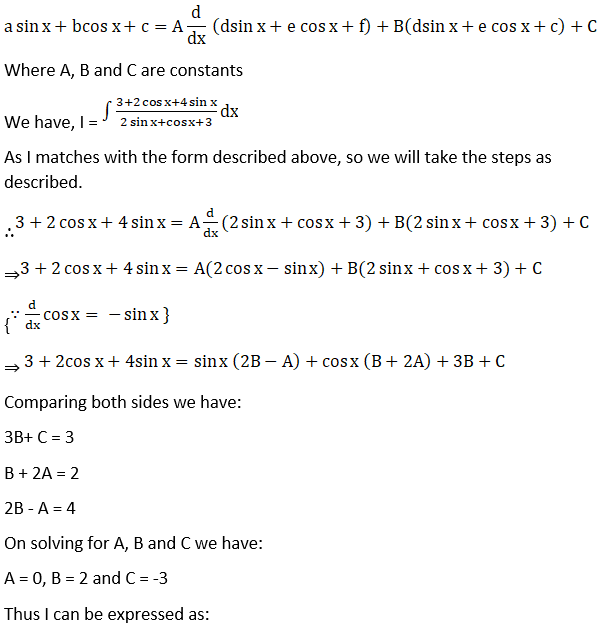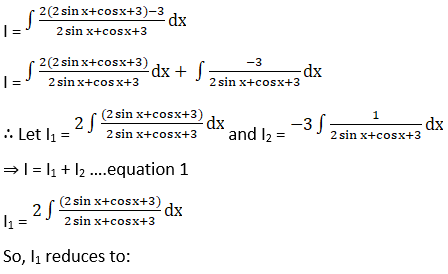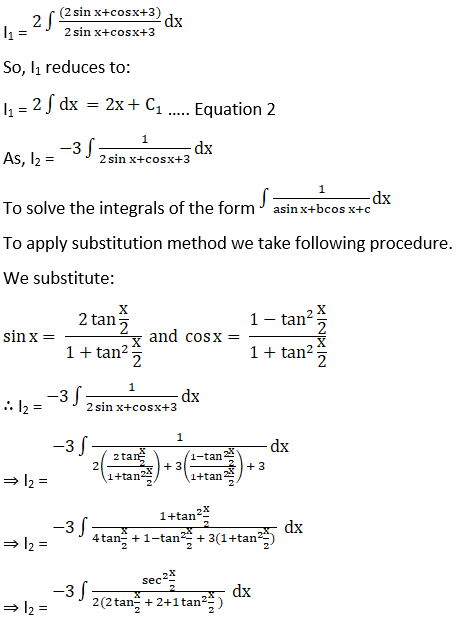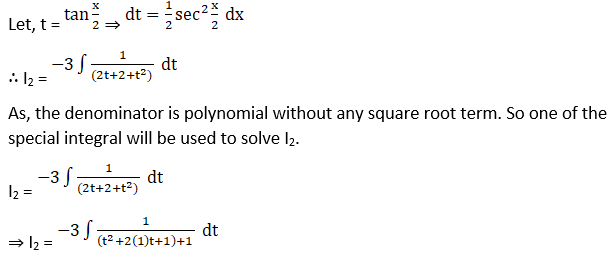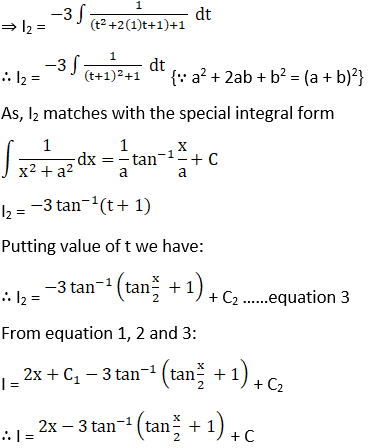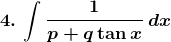Solution: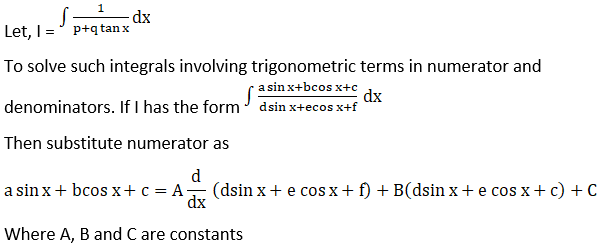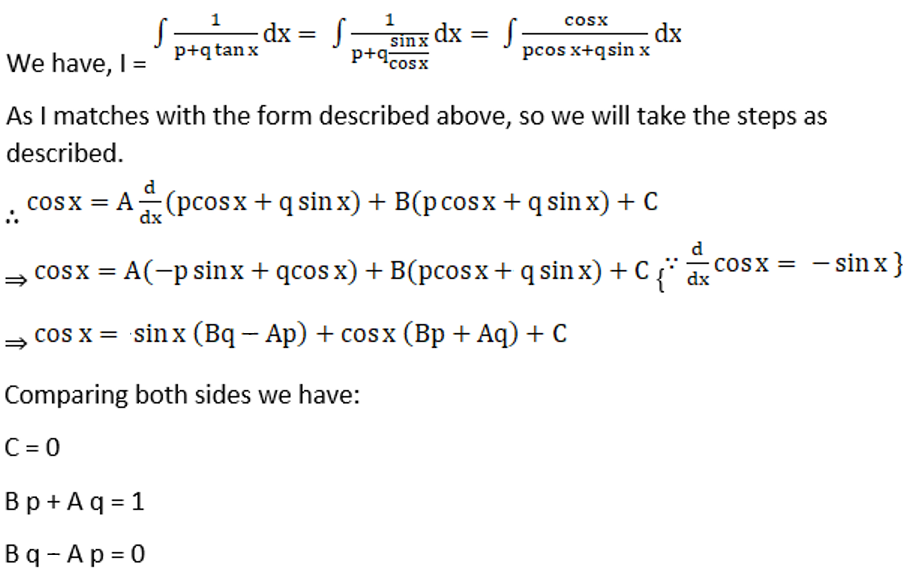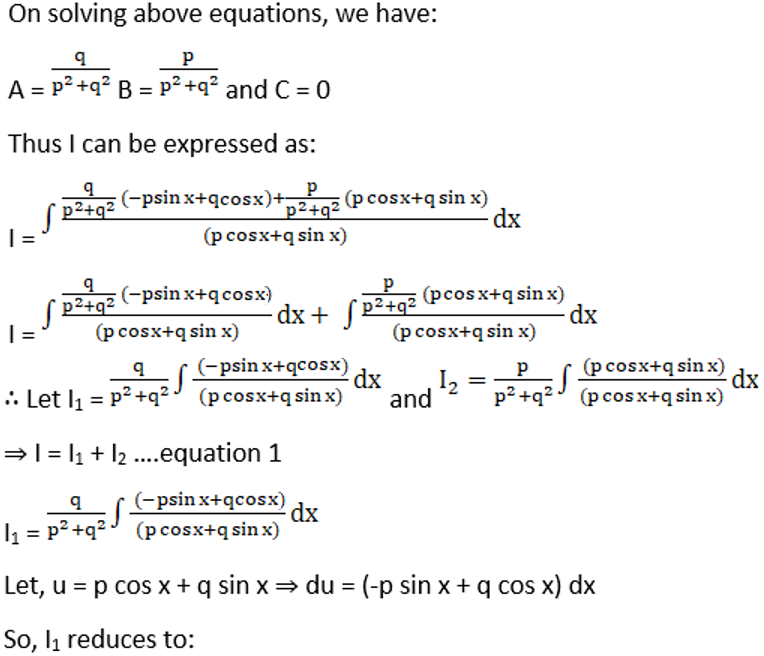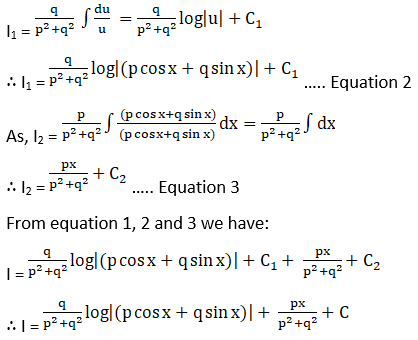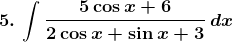Solution: